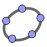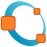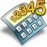Mathematical Software for Mac

Enter the fascinating world of maths and discover a wide range of software for Mac to solve all sorts of problems, equations and calculations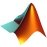MATLAB R2019a

The most renowned maths software in the world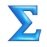MathType 7.4.2

Equation editor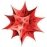Mathematica 12.0.0

Computational software for Mac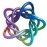Maple 2018.1 Update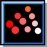Scilab 6.0.2